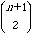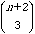Math 497 Notes January 24, 2002

Homework Discussion

Problem 1.1 postponed until next week.

## The Sphere Problem

A great circle divides a sphere into 2 hemispheres. One special property of a great circle is that any two great circles intersect in two points (on opposite positions on the sphere). Use a ball and rubber bands to approximate a sphere and great circles. [Todd brought some great foam balls for this.]

For n = 1, 2, 3, 4, 5, 6, great circles, see how many pieces the sphere is cut into by n great circles. Make a table of your results:

A1 = 2

A2 = 4

A3 = 8

A4 = 14

A5 = 22

Then conjecture a formula if you can. After this, use the difference table method to find a formula and check the formula on the next case or two.

1. Joey's answer was a recursive pattern. He found this by taking differences between entries in the table, which are 2, 4, 6, 8, … and recognizing this pattern at 2(n-1).

An = An-1 + 2(n-1)

2. Roger found this formula for An: An = n2-n+2. His method involved noticing that the numbers seem to be going up like n and then taking the difference and noticing the pattern.

A1 - 12 = 2 - 1 = 1

A2 - 22 = 4 - 4 = 0

A3 - 32 = 8 - 9 = -1

A4 - 42 = 14 - 16 = -2

A5 - 52 = 22 - 25 = -3

3. Someone (Todd?) looked at a calculus book and looked for a common formula. This was a fine start to a long conversation about this problem. There were two main points:
• How do we know by how much the number of pieces is increased by adding one great circle to, say, 83 circles already drawn on the circle? For such large numbers it is impractical to count accurately, and of course we can't count all possible cases. This is important, since in the previous class we saw how apparent patterns can break down after a number of terms. For math, we need to reason for all cases.

Once we have the pattern. How can we find a formula for the number of pieces cut out by n circles?

Note: The number of pieces is a not always the same. For example, for 3 circles, the number of pieces is 6 if all 3 circles pass through the North Pole and the South Pole. But if the 3 circles do not pass through any single point, the number is 8. The number we are seeking is for the "general case". This is the case when no great circle passes through a point of intersection of other circles. It will occur if the circles are "random." This will produce the maximum number of regions on the sphere. (The minimum number occurs when all the circles pass through the same two points.) Adding a new circle means finding a great circle that does not go thru any other common point of intersection.

Reasoning for the number of new regions when adding a circle. For each great circle you add, you cross each of the other great circles twice. This means you cross n-1 great circles with the nth new band at 2(n-1) places. It also means you create an arc every time you cross a new great circle or you create 2(n-1) new arcs and those arcs divide each region into two pieces. So you are adding 2(n-1) new regions to the regions you had before. This justifies Joey's recursive formula. As Roger pointed out, it is important that each new circle crosses each of the old circles at two points, but it does NOT cross all the regions.

How to discover the formula and be sure of it? Here are some approaches., starting with tricks and insights and moving up to general methods.

Method 1. Guess the formula and then check it by taking differences. This means that if we assume Roger's formula An = n2-n+2. The problem with this is how to guess the formula.

An+1 — An = [(n+1)2—(n+1)+2] —(n2-n+2) = 2(n+1) - 2

Method 2. Recognize connection with triangular numbers. These differences are the same as double the differences for triangular numbers, so the numbers of regions equals double the triangular numbers plus a constant.

Method 3. Use the general Newton method that uses difference tables. This is related to Pascal's triangle. More explanation may be forthcoming later, but until then, there is a rather explicit description of the method on pages 81- 82, with a generalization on pages 82-83. YOU SHOULD WORK OUT THE EXAMPLE WITH THIS METHOD.

In class, it was pointed out that Pascal's triangle can be viewed as a difference scheme (by tilting it on its side). There may be more details posted later.

### Pascal's triangle

1

1 1

1 2 1

1 3 3 1

1 4 6 4 1

1 5 10 10 5 1

1 6 15 20 15 6 1

First diagonal = F0 (n) = 1

Second diagonal = F1(n) = n

Third diagonal = F2(n) = [n(n+1)]/2 =Fourth diagonal = F3(n) = [n(n+1)(n+2)]/6 =## Pascal Triangle Problem

We all had colored in the triangle, with odd number black and even numbers white. Then this gives a fractal program. The question is why is this true.

1

1 1

1 2 1

1 3 3 1

1 4 6 4 1

1 5 10 10 5 1

1 6 15 20 15 6 1

1 7 21 35 35 21 7 1

1 8 28 56 70 56 28 8 1

Secret: If you calculate "mod 2" this means that each number is represented by the symbol 0 for even numbers and 1 for odd numbers, and then regular addition and multiplication holds.

Then the Pascal theorem looks like this mod 2.

1

1 1

1 0 1

1 1 1 1

1 0 0 0 1

1 1 0 0 1 1

1 0 1 0 1 0 1

1 1 1 1 1 1 1 1

1 0 0 0 0 0 0 0 1

Recall the Pascal coefficients are the binomial coefficients of the expansion of (x+y)n. So you can read the coefficients mod 2 from the triangle.

Consider what (x + y)2 looks like mod 2. Corresponds to row 1 0 1.

Since for integers, (x + y)2 = x2 + 2xy + y2, this number is the same mod 2 as x2 + y2 since 2xy must be even and this is 0 mod 2.

This explains what happens to other rows if we can square and square again. For example

((x + y)2 )2 = (x2 + y2)2 = (x2)2 + (y2)2 = x4+ y4.

To be more systematic:

If n is a power of 2 such as 2, 4, 8, 16, … , then (x + y)n = xn + yn mod 2.

This means that the corresponding rows in the triangle are rows of all zeros except for the 1's at the ends. You can see this in the triangle above for n = 2, 4, 8.

We continued looking at these patterns by looking at the Pascal triangle mod 3 and mod 4. We found the simple fractal pattern occurs when the modulus is a prime p such as 3 but not for 4, because.

If p is prime, and if n is a power of p, then (x + y)n = xn + yn mod p.